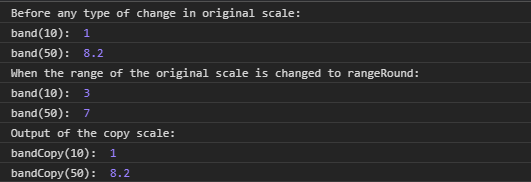# D3.js band.copy() Function

The band.copy() function is used to construct and return the copy of the current scale with the same domain and range. Any changes made to any scale are independent of each other i.e change in original scale will not affect the copy scale and vice-versa.

Syntax:

`band.copy();`

Parameters: This function does not accept any parameter.

Return Values: This function returns a copy of the current scale. The return type is a function.

Below given are a few examples of the function given above.

Example 1:

 `  ` `<``html` `lang` `= ``"en"``>  ` `<``head``>  ` `    ``<``meta` `charset` `= ``"UTF-8"` `/>  ` `    ``<``meta` `name` `= ``"viewport"` `        ``path1tent = "``width``=``device``-width,  ` `        ``initial-scale` `= ``1``.0"/>  ` `    ``<``script` `src` `= ` `    ``"https://d3js.org/d3.v4.min.js"``> ` `    `` ` `     `  `  ` `<``body``>  ` `    ``<``script``>  ` `    ``// Creating the band scale  ` `    ``//with specified domain and range. ` `        ``var band = d3.scaleBand() ` `                    ``.domain([10, 20, 30, 40, 50]) ` `                    ``.range([10, 100]); ` `    ``// Discrete values are automatically created  ` `    ``// By the band function ` `    ``// Band of the range is [10, 100] ` `        ``console.log("band(10): ", band(10)); ` `        ``console.log("band(50): ", band(50)); ` ` `  `        ``var bandCopy = band.copy(); ` `        ``console.log("bandCopy(10): ", bandCopy(10)); ` `        ``console.log("bandCopy(50): ", bandCopy(50)); ` `    ``  ` `  ` ``

Output:Example 2:

 `  ` `<``html` `lang` `= ``"en"``>  ` `<``head``>  ` `    ``<``meta` `charset` `= ``"UTF-8"` `/>  ` `    ``<``meta` `name` `= ``"viewport"` `        ``path1tent = "``width``=``device``-width,  ` `        ``initial-scale` `= ``1``.0"/>  ` `    ``<``title``>GeekforGeeks  ` `    ``<``script` `src` `= ` `    ``"https://d3js.org/d3.v4.min.js"``> ` `    `` ` `     `  `  ` `<``body``>  ` `    ``<``script``>  ` `    ``// Creating the band scale ` `  ``// with specified domain and range. ` `        ``var band = d3.scaleBand() ` `                ``.domain([10, 20, 30, 40, 50]) ` `                ``.range([1, 10]); ` `// Discrete values are automatically created  ` `    ``// By the band function ` `    ``// Band of the range is [1, 10] ` `        ``console.log( ` `"Before any type of change in original scale:"); ` `        ``console.log("band(10): ", band(10)); ` `        ``console.log("band(50): ", band(50)); ` `        ``// Making copy of the original scale ` `        ``var bandCopy = band.copy(); ` `        ``console.log( ` `"When the range of the original "+ ` `"scale is changed to rangeRound:"); ` `        ``// Making changes to the original scale ` `        ``band.rangeRound([1, 10]); ` `        ``console.log("band(10): ", band(10)); ` `        ``console.log("band(50): ", band(50)); ` `        ``console.log("Output of the copy scale:"); ` `        ``console.log("bandCopy(10): ", bandCopy(10)); ` `        ``console.log("bandCopy(50): ", bandCopy(50)); ` `    ``  ` `  ` ``

Output:My Personal Notes arrow_drop_upCheck out this Author's contributed articles.

If you like GeeksforGeeks and would like to contribute, you can also write an article using contribute.geeksforgeeks.org or mail your article to contribute@geeksforgeeks.org. See your article appearing on the GeeksforGeeks main page and help other Geeks.

Please Improve this article if you find anything incorrect by clicking on the "Improve Article" button below.

Article Tags :

Be the First to upvote.

Please write to us at contribute@geeksforgeeks.org to report any issue with the above content.